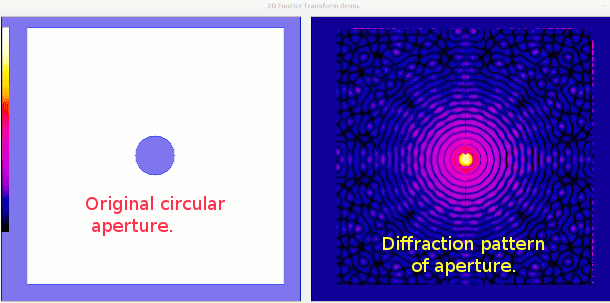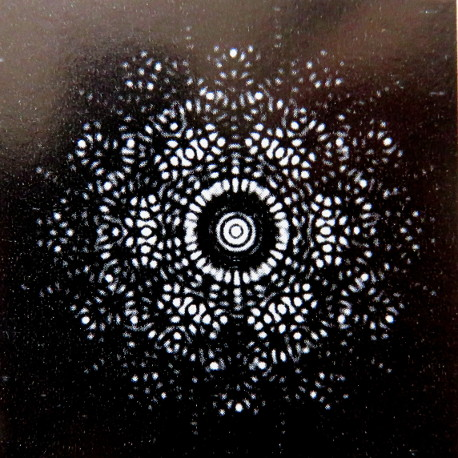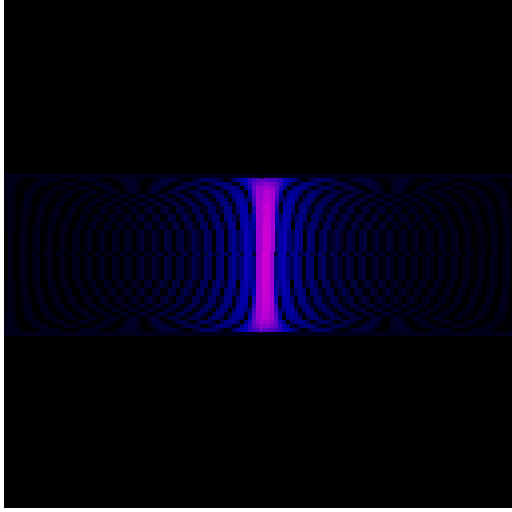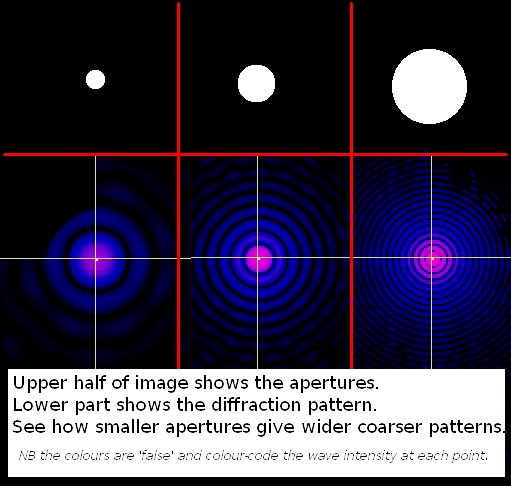# 2-Dimensional Diffraction- FFT in 2D## What is optical diffraction?

If you shine a monochromatic light beam- such as a laser- through a pattern of one or more holes, obstacles, slits, etc, the light behave like a wave and does not give a 'sharp shadow' but spreads and creates an 'interference pattern'. ( Part of the mystery of modern physics is this ability to be both wave and particle..)In my student days we didn't have lasers to give coherent monochromatis light. It was still magic to be able to flip from frequency domain to real world. The beauty of the images, and the rapidly developing application of X-rays to crystallography were very attractive to me. A year or two later and I built a mains powered He-Ne gas laser. Now we can buy high-intensity solid-state laser cheaply on the 'net.## What is a ( 1D) Fourier Transform??

A Fourier Transform is a bit of maths that turns a sample of a waveform repeating on one axis- often time- into a collection of sine and cosine terms that are a close approximation to the original and can be used to generate a continuous sample of the original 'waveform'. Basically you see how much of a series of faster and faster waves is needed at each frequency, multiples of the fundamental frequency. This is slow to calculate, and gets SERIOUSLY slower as you increase the number of samples- order N-squared +.

The screen shots below show the analysis and re-synthesis of four waves.

Beats shows 'sin 30 +sin 33'. Pulse is a low mark/space rectangular wave. Square wave approximation was generated from the known series of terms.. .. .Example LB code- uses a supplied colour-scale.

```

'   ***********************************************************
'   **                                                       **
'   **  1DFFT.bas       27/02/2017       tenochtitlanuk      **
'   **                    beats                              **
'   ***********************************************************

global n, pi                    '   globally available pi, number of data values- a multiple of 2-

n       =1024
'   globally available real and imaginary data for main and sub sections
dim realData( n), imagData( n)  '   the FFT operates in-place, changing the data to its transform.

pi      =   4 *atn( 1)

WindowWidth  =1080
WindowHeight = 720

nomainwin

open "FFT demo." for graphics_nsb as #wg

#wg "trapclose quit"
#wg "goto 20 100 ; down ; size 1 ; fill cyan ; color white"

for i =1 to n
'if i >508 and i <516 then realData( i)  =1 else realData( i)  =0.05
for k = 1 to 3 step 2
realData( i) =realData( i) +1 /k *sin( i /n *k *10 *2 *pi)
next k
imagData( i)  =0
#wg "color red      ; line "; 20 +1024 *i /n; " 80 "; 20 +1024 *i /n; " "; 80 -int( 25 *realData( i))
next i

call fft -1 '   ie compute FFT not inverse-   1 for fwd FFT, -1 for reverse

#wg "size 5"
for i =1 to n ' but symmetrical on x axis so really only want left-hand half.
#wg "color red      ; line "; 20 +1024 *i /n; " 250 "; 20 +1024 *i /n; " "; 250 -int( 0.18 *realData( i))
#wg "color darkblue ; line "; 20 +1024 *i /n; " 350 "; 20 +1024 *i /n; " "; 350 -int( 0.18 *imagData( i))
next i

call fft 1  '   ie compute inverse

#wg "size 1"
for i =1 to n ' but symmetrical on x axis so really only want left-hand half.
#wg "color red      ; line "; 20 +1024 *i /n; " 550 "; 20 +1024 *i /n; " "; 550 -int( 25 *realData( i))
next i

#wg "color black ; up ; goto 10 80 ; down ; goto 1034 80"

for v =250 to 550 step 100
if v <>450 then #wg "up ; goto 10 "; v; " ; down ; goto 1034 "; v
next v

wait

sub fft isi
j   =1
for i =1 to n
if i <j then call exchangeTerms i, j
m   =n /2
while j >m
j   =j -m
m   =int( ( m +1) /2)
wend
j   =j +m
next i

mmax    =1

while mmax <n
istep   =2 *mmax
for m =1 to mmax
theta   =pi *isi *( m -1) /mmax
wr      =cos( theta)
wi      =sin( theta)

for i =m to n step istep
j               =i +mmax
tempr           =wr *realData( j) -wi *imagData( j)
tempi           =wr *imagData( j) +wi *realData( j)
realData( j)    =realData( i) -tempr
imagData( j)    =imagData( i) -tempi
realData( i)    =realData( i) +tempr
imagData( i)    =imagData( i) +tempi
scan
next i
next m

mmax    =istep
wend

if isi =-1 then exit sub    '   else normalise values

for i =1 to n
realData( i)  =realData( i) /n
imagData( i)  =imagData( i) /n
next i
end sub

sub exchangeTerms i, j
temp            =realData( i)
realData( i)    =realData( j)
realData( j)    =temp

temp            =imagData( i)
imagData( i)    =imagData( j)
imagData( j)    =temp
end sub

sub quit h\$
close #h\$
end
end sub
```
..which needs this colour-scale bmp saved in the same directory folder.## What is a 2D Fourier Transform?

If the input data is 2D instead of 1D, you first transform each ROW and then in a second pass transform each COLUMN. The data is kept in-situ throughout.

## What is a Fast Fourier Transform??

A FFT is a way of implementing the transform in a reasonable time!Starting with a circular aperture the result of the two series of 1D transforms is the 2D result shown. Note that to agree with the way we see diffraction the results need re-assembling by re-ordering the four quarters, as done below . . .. . .```

'                       ***********************************************************
'                       **                                                       **
'                       **   2DFourier9.bas       05/03/2017     tenochtitlanuk  **
'                       **                       beats                           **
'                       ***********************************************************

nomainwin

global n, pi                    '   globally a<ailable pi, number of data <alues- a multiple of 2-
n            = 128
'   globally a<ailable real and imaginary data for main and sub sections
dim realData( n), imagData( n)  '   the FFT operates in-place, changing the data array to its transform.
dim imageArray\$( n, n)          '   holds R and I <alues as a cs< string
dim ColLookUp\$( 256)

pi           =   4 *atn( 1)

UpperLeftX   =  10
UpperLeftY   =  10
WindowWidth  =1300
WindowHeight = 700

graphicbox #w.gb1,   20, 10, 600, 570
graphicbox #w.gb2,  640, 10, 600, 570

open "2D Fourier Transform demo." for window as #w

#w     "trapclose quit"
#w.gb1 "down ; fill 40 40 180 ; size 1 ; color darkgray"
#w.gb2 "down ; fill 40 40  40 ; size 1 ; color darkgray"

#w.gb1 "drawbmp scr 0 20 ; size 1"

gosub [fillColLookUp]
gosub [initImageArray]

for row =1 to n
for col =1 to n   '   copy a row of data to array for computing its FFT
realData( col)    =<al( word\$( imageArray\$( row, col), 1, ","))
imagData( col)    =<al( word\$( imageArray\$( row, col), 2, ","))
next col

call fft -1

for col =1 to n   '   do FFT and copy back to image array
imageArray\$( row, col)   =str\$( realData( col)) +"," +str\$( imagData( col))
amp                      =amp( ( realData( col)^2 +imagData( col)^2)^0.5)      '   int( 100 *log( 1 +( realData( col)^2 +imagData( col)^2)^0.5))
#w.gb2 "backcolor "; ColLookUp\$( amp)
#w.gb2 "color     "; ColLookUp\$( amp)
call sqDraw 2, row, col
next col

next row

call screenGrab "X"

for col =1 to n
for row =1 to n         '   copy a row of data to array for computing its FFT
realData( row)          =<al( word\$( imageArray\$( row, col), 1, ","))
imagData( row)          =<al( word\$( imageArray\$( row, col), 2, ","))
next row

call fft -1

for row =1 to n
imageArray\$( row, col)   =str\$( realData( row)) +"," +str\$( imagData( row))
amp                      =amp( ( realData( row)^2 +imagData( row)^2)^0.5)      '   int( 100 *log( 1 +( realData( row)^2 +imagData( row)^2)^0.5))
#w.gb2 "backcolor "; ColLookUp\$( amp)
#w.gb2 "color     "; ColLookUp\$( amp)
call sqDraw 2, row, col
next row

next col

'call screenGrab "Y"
wait

sub screenGrab i\$
for x =0 to 256 step 256
for y =0 to 256 step 256
#w.gb2 "getbmp scr "; x +50; " "; y +20; " 256 256"
bmpsa<e "scr", i\$ +str\$( x) +str\$( y) +".bmp"
next y
next x
end sub

sub fft isi
j   =1
for i =1 to n
if i

Code will be up shortly as a 2D_FFT.zip

```